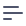# Using Dynamic Measures

## Use case

We want to understand the distribution of orders by their statuses. Let's imagine that new order statuses can be added in the future, or we get a list of statuses from an external API. To calculate the orders percentage distribution, we need to create several measures that refer to each other. But we don't want to manually change the schema for each new status. To solve this, we will create a schema dynamically.

## Data schema

To calculate the number of orders as a percentage, we need to know the total number of orders and the number of orders with the desired status. We'll create two measures for this. To calculate a percentage, we'll create a measure that refers to another measure.

const statuses = ['processing', 'shipped', 'completed'];

const createTotalByStatusMeasure = (status) => ({
[Total_${status}_orders]: { type: count, title: Total${status} orders,
filters: [
{
sql: (CUBE) => ${CUBE}."status" = '${status}',
},
],
},
});

const createPercentageMeasure = (status) => ({
[Percentage_of_${status}]: { type: number, format: percent, title: Percentage of${status} orders,
sql: (CUBE) =>
ROUND(${CUBE[Total_${status}_orders]}::numeric / \${
CUBE.totalOrders
}::numeric * 100.0, 2),
},
});

cube(Orders, {
sql: SELECT * FROM public.orders,

measures: Object.assign(
{
totalOrders: {
type: count,
title: Total orders,
},
},
statuses.reduce(
(all, status) => ({
...all,
...createTotalByStatusMeasure(status),
...createPercentageMeasure(status),
}),
{}
)
),
});

## Result

Using the measures defined above, we can explore the orders percentage distribution and easily create new measures just by adding a new status.

[
{
'Orders.Percentage_of_processing': '33.54',
'Orders.Percentage_of_shipped': '33.00',
'Orders.Percentage_of_completed': '33.46',
},
];

## Source code

Please feel free to check out the full source code or run it with the docker-compose up command. You'll see the result, including queried data, in the console.